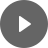13.1 Simple Interest - StudyNinja
Select Page

HSC Standard Maths Resources

Browse: 1. Home  »  13. Investments » 13.1 Simple Interest

13.1 Simple Interest

Simple Interest, as the name suggests, is quite simple to work with and understand. The following is the formula to calculate interest:When answering questions, there is one thing you need to be careful about that the interest rate and time in the above formula are in the same frequency i.e. if interest rate is given ‘per annum’ then time should also be in years. If they are not, then convert them before applying formula. It doesn’t matter which one you convert as long as you make them same the frequency. It is also not just the interest amount, you can be asked to calculate principal, interest rate or time.

Example 1

How much would be interest on an investment in 25 months which is worth \$4,500 today if it earns simple interest at 3.65% p.a.?

Example 2

An investment earns \$8,575 total interest in 7 years where simple interest rate is 1.225% per quarter. What is the amount of investment?

Example 3

An investment earns \$1,955 total interest in 5 years on a principal of \$17,000. What is the simple interest rate earned per annum?

Get access to 20 Mock Exams with over 700 exam-style questions for HSC Standard Maths.

You might find the following videos helpful related to this section:3B Interest Graphs (1 of 2)

by Mr Bodgers (click to view channel)

Explains how to represent a simple interest problem as a graphThe following is the type of questions you can expect in exam:

Study notes of this section and other resources can be accessed here:

Browse: 1. Home  »  13. Investments » 13.1 Simple Interest